## Drawing Curves

All of Omber's shapes and lines are made up of lines segments. These segments can be straight or curved. In Omber, all curved segments are Bezier curves.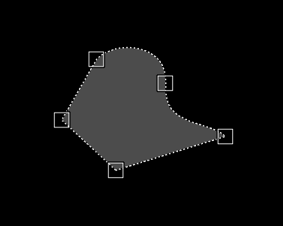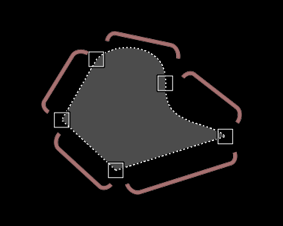When drawing shapes, you can switch between "straight" mode and "curve" mode to draw the straight and curved sections of your shapes. To choose which mode to use, you can click on the "straight" or "curve" buttons in the context panel at the top of the screen.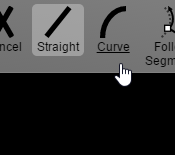When in "Curve" mode, Omber will try to draw a smooth curve between the last segment and the current position. If you are drawing the first segment of a shape, there is no previous segment, so Omber will simply show a straight line between the two points.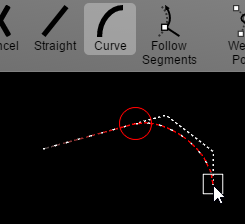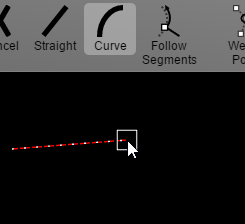After setting the two endpoints of the curve, Omber lets you adjust the shapes of the curve. The two bezier handles that dictate the shape of the curve will be shown, and you can drag the handles to change the curve's shape.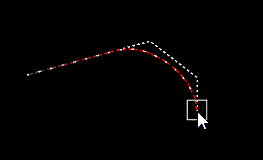Once you are satisfied with the shape of the curve, you can then draw the next curved segment of the shape by adding a new endpoint like before.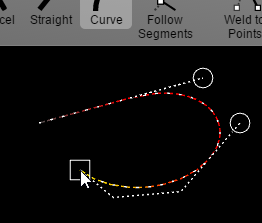### Matching Curve Ends To Be Smooth

When making shapes, you often need to join together multiple simple curves to make a more complex curve. It can be unclear how to make the transitions between curves appear to be smooth when joining them together.Omber uses four-point Bezier curves for its curved segments. Bezier curves are popular in digital art because they give you control over the angles at its end-points. The angle formed between a Bezier curve's control point and its end-point will be the same as the angle of the curve at that end-point.To have two curves join smoothly together, you want the angle of the curves to match at the point where they join. To get the angles of the curves on either side of the join point to match, you should align the control points on either side of the join point so that they form a straight line. This ensures that the angles of both curves are the same at the join point.It's possible to make the transition between the two curves even smoother. It's possible to draw a line from the join point to the control point of the curve. If the lines of the control points on either side of the join point are the same length, then the join between the curves will seem very smooth.When working with curves, Omber will display guidelines to help you place control points that result in smooth curve transitions. If a curve already has a smooth transition with other curves, you can hold down the shift key or the Multi button while dragging the curve's handles to force other curves to change shape to maintain this smooth curve transition.

Contents